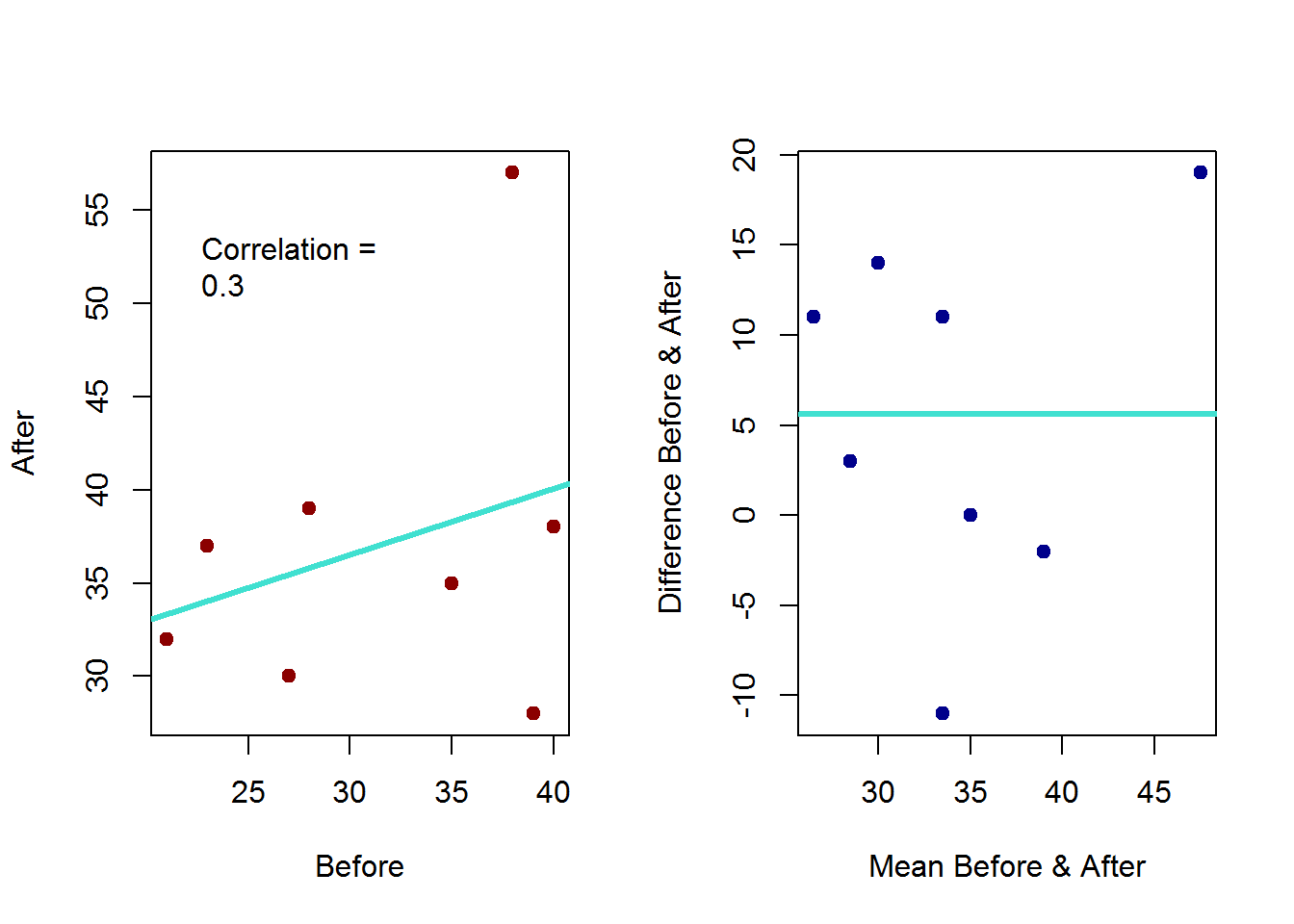### PAIRED $$t$$ TEST:

We can compare the difference in mean between paired observations by just focusing on the whether the difference is consistent with zero as though it was a single group: $$H_o: \mu_{\text{diff}} = 0$$ and $$H_a: \mu_{\text{diff}} \neq 0$$.

In this case the $$t$$ statistic is:

$\text{test statistic}=\frac{\bar X_{\text{diff}} - \,0}{SD_{\text{diff}}\,\sqrt{n_{\text{diff}}}} \geq \,\text{qt}\,(0.95,\, n_{\text{diff}} - 1)$

Of note, the standard error of the difference in pair data is:

$\sqrt{\frac{\sigma_x^2}{n}+\frac{\sigma_y^2}{n}-2\frac{\text{cov}(X,Y)}{n}}$

Of course, for a large sample we could also use a z statistic.

It’s a good thing for paired data to get the **Mean-Difference Plot“:

dat <- read.csv("dat.csv", header=T)
attach(dat)
dat
##   Student Before After
## 1       1     21    32
## 2       2     35    35
## 3       3     40    38
## 4       4     38    57
## 5       5     23    37
## 6       6     27    30
## 7       7     28    39
## 8       8     39    28
mean <- (Before + After)/2
diff <- After - Before
par(mfrow=c(1,2))
plot(After ~ Before, pch= 19, col= "red4")
corr <- round(cor(Before, After), 2)
legend(x = 20, y = 55, legend=c("Correlation =",corr), bty="n")
abline(lm(After ~ Before), lwd = 3, col= "turquoise")
plot(mean, diff, pch = 19, col= "blue4", xlab = "Mean Before & After", ylab = "Difference Before & After")
abline(h=mean(diff), col="turquoise", lwd = 3)Now doing the calculations for this dataset:

diff <- After - Before
n <- sum(!is.na(diff))
mean(diff)
sd(diff)
(test_statistic <- sqrt(n) * mean(diff) / sd(diff))
##  1.614366
# Cut-off limit:

c(-1, 1) * qt(0.975, n - 1)
##  -2.364624  2.364624
# p value:

2 * pt(abs(test_statistic), n - 1, lower.tail = FALSE)
##  0.1504825

A not so significant difference!

Compare to:

t.test(diff)
##
##  One Sample t-test
##
## data:  diff
## t = 1.6144, df = 7, p-value = 0.1505
## alternative hypothesis: true mean is not equal to 0
## 95 percent confidence interval:
##  -2.614155 13.864155
## sample estimates:
## mean of x
##     5.625